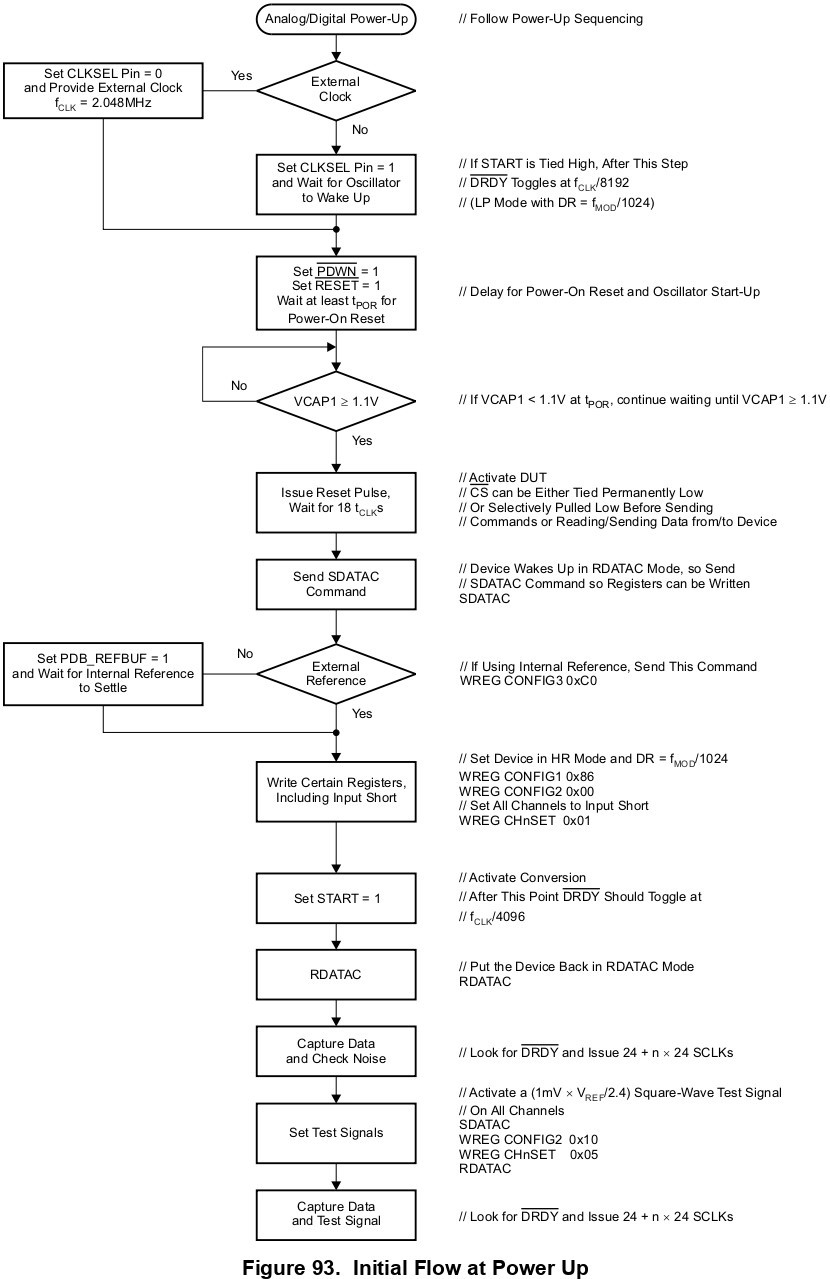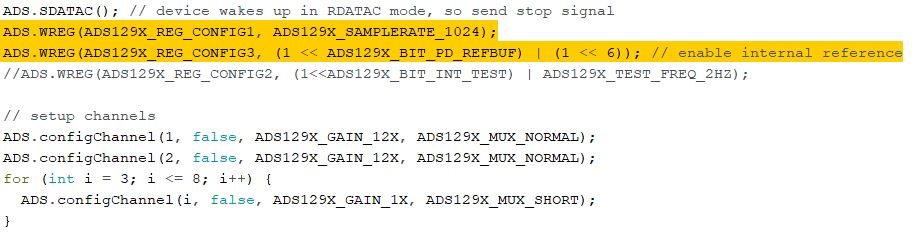If you have a related question, please click the "Ask a related question" button in the top right corner. The newly created question will be automatically linked to this question.

Hello TI Community,

I have been playing around with ADS1298 for ECG and EMG acquisition.

I have made a custom PCB that interfaces ADS1298 and Teensy 3.2 (using the Arduino interface).

I have followed the following flowchart given in the ADS1298 datasheet.The settings that I have chosen are as follows:

• LP mode
• DR = 250sps
• I have attached the registers settings below:I have also checked the DRDY signal, again attached below:Also, I have tested the IC using an internal test signal (Channel 1 and 2 are kept at 12X gain whereas the Rest of the channels 3 to 8 are kept at a 1X gain):Also, I checked the internal voltage nodes as you have available on my device:

• VREF = VREFP – VREFN = (0- (-2.5V)) = 2.5V
• VCAP1 = AVSS + 1.2 V = -2.5 + 1.2 = -1.3V
• VCAP2 = (AVDD + AVSS) / 2 = (2.5-2.5) / 2 = 0V
• VCAP3 = AVDD + 1.9 V = 2.5 + 1.9 = 4.4V
• VCAP4 = (VREFP + VREFN) / 2 = (0 - 2.5)/ 2 = -1.25V
• The above internal voltage node values are calculated and when I probe these nodes I get the same values.

SO the issue I am facing is as follows:

• I have connected the electrode cables at IN1P and IN1N (And similarly for the rest of the inputs 2-8 channels) and placed these electrodes on my forearm in order to extract the differential signal (in EMG form). I am not getting the EMG burst signal when I flex my muscle. What could be the issue?
• Can you check my register configuration? The SPI is working since I am able to read back the register after writing them.
• What should be the configuration to set (Not necessarily optimum one but a rough idea) in order to acquire ECG and EMG signals?
• Also, I might have many follow-up questions too.

PS: DO not forward me another discussion thread

• Hi Moin,

Thanks for the post.

There seems to be a lot of powerline noise in your internal test signal plot. Can you please look at the measurement when the input is shorted to the common-mode voltage? It may be helpful to look at the baseline EMG measurement and compare it to the measurement with muscle activity. Are you using the RLD to improve the CMRR and also to bias the body? The device configuration seems fine for EMG signals (LP, 250SPS).

• VREF = VREFP – VREFN = (0- (-2.5V)) = 2.5V
• VCAP1 = AVSS + 1.2 V = -2.5 + 1.2 = -1.3V
• VCAP2 = (AVDD + AVSS) / 2 = (2.5-2.5) / 2 = 0V
• VCAP3 = AVDD + 1.9 V = 2.5 + 1.9 = 4.4V
• VCAP4 = (VREFP + VREFN) / 2 = (0 - 2.5)/ 2 = -1.25V
• The above internal voltage node values are calculated and when I probe these nodes I get the same values.

Can you confirm you design with VREFP = 0V and VREFN= -2.5V?

Thanks

-TC